### Home > PC > Chapter 4 > Lesson 4.2.2 > Problem4-84

4-84.
1. Find the specified value given the information provided. Assume that 0 ≤ θ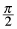. Homework Help ✎

1. csc θ =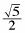; find sin θ

2. tan θ = 0.8; find cot θ

3. cos θ =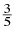; find sin θ

4. csc θ =; find cosθ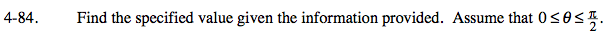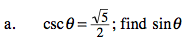$\text{sin}\theta=\frac{1}{\text{csc}\theta}$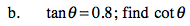$\text{Rewrite }(.8)\text{ as }\frac{8}{10}.\text{ The cot}\theta=\frac{1}{\text{tan}\theta}.$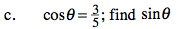1. Draw and label a sketch.
2. Find the third side.
3. Find the sin θ.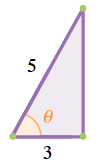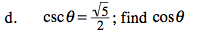Follow the same steps as (c) above.
Be careful labeling the sides of the triangle!